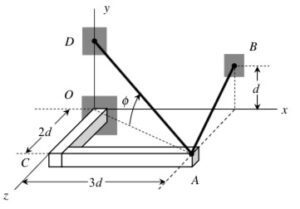# Homework H2.B - Sp23

 Problem statement (Updated Tues, Jan 10th @ 10:42am) Solution videoPlease post questions here on the homework, and take time to answer questions posted by others. You can learn both ways.

## 10 thoughts on “Homework H2.B - Sp23”

1.StaticsW27 says:

Can someone explain how to find the unit vector on part b because the position vector is composed of both variables and numbers?

1.Samuel Aldridge says:

I believe for this problem you can use the direction angles strategy to find the unit vector. The first example from Period 2 shows how this can be done.

2.MiKayla M Beals says:

In what notes do we talk about the resultant and how to find it?

1.Connor Chadwick says:

I don't think they do but I am pretty sure you find it by adding the force vectors

1.Lily Krest (TA) says:

This is correct, the resultant (which is a vector) is just the sum of the force vectors.

3.MiKayla M Beals says:

How do we find direction cosines and direction angles with the d being in there since they don't cancel out?

1.MiKayla M Beals says:

nevermind I figured out where they cancel

4.Min says:

On part b (finding unit vector), I used Φ direction angle to find out the projection of F(AD) onto the xz plane. And I noticed that there is no additional θ or other degree sign that i can use to figure out the unit vector for x,y,z...

Do i have to use only Φ direction angle to figure out the unit vector for x,y,z?

1.Min says:

In the first example of 2nd period, there were two angle in the figure : Φ and θ...

2.Lily Krest (TA) says:

The process I've been suggesting to students is to start with finding the length DO so that you can write the coordinates of point D. After you find that length you can do A-D to get the position vector, and use that to get the unit vector. This isn't the only way to do this problem, but it's a process that will always be applicable when finding a unit vector between two points.